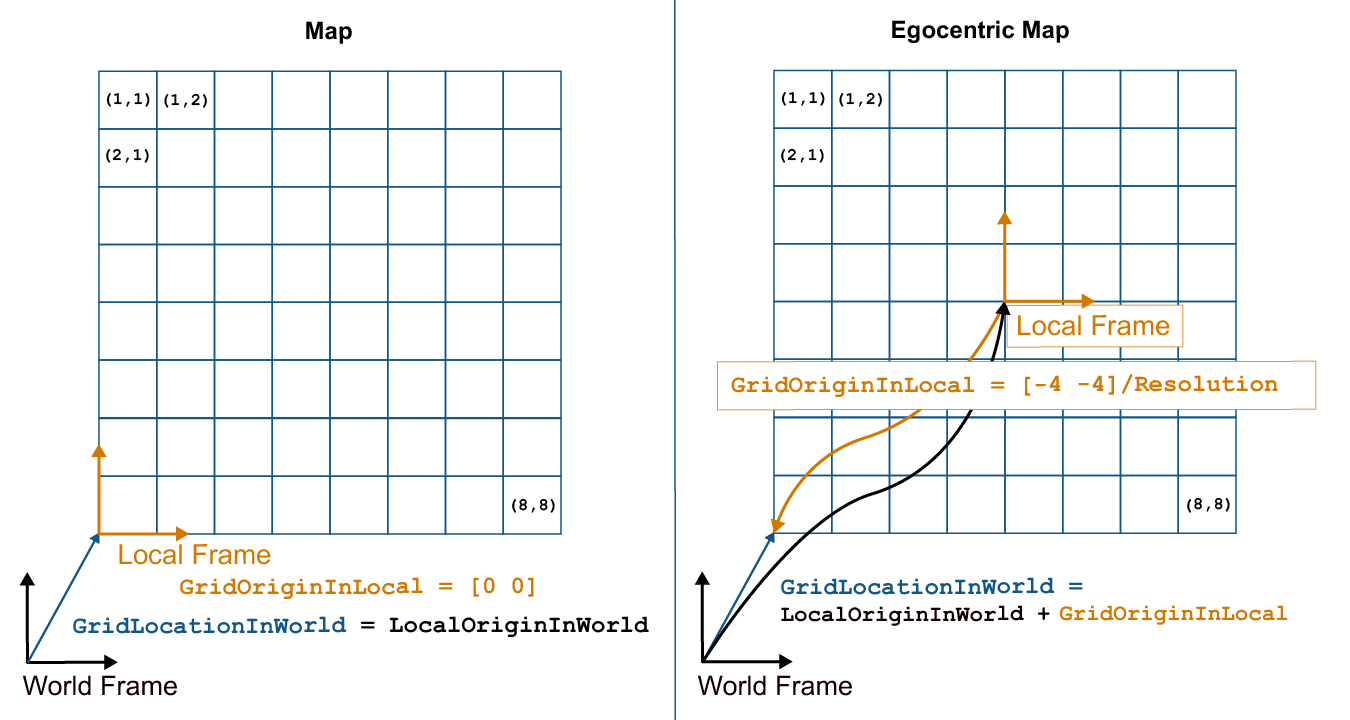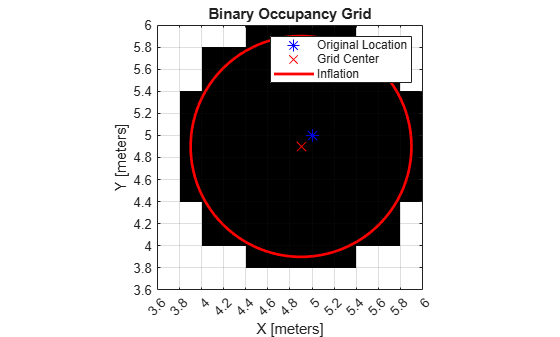## Occupancy Grids

### Overview

Occupancy grids are used to represent a robot workspace as a discrete grid. Information about the environment can be collected from sensors in real time or be loaded from prior knowledge. Laser range finders, bump sensors, cameras, and depth sensors are commonly used to find obstacles in your robot’s environment.

Occupancy grids are used in robotics algorithms such as path planning (see `mobileRobotPRM` or `plannerRRT`). They are used in mapping applications for integrating sensor information in a discrete map, in path planning for finding collision-free paths, and for localizing robots in a known environment (see `monteCarloLocalization` or `matchScans`). You can create maps with different sizes and resolutions to fit your specific application.

For 3-D occupancy maps, see `occupancyMap3D`.

For 2-D occupancy grids, there are two representations:

A binary occupancy grid uses `true` values to represent the occupied workspace (obstacles) and `false` values to represent the free workspace. This grid shows where obstacles are and whether a robot can move through that space. Use a binary occupancy grid if memory size is a factor in your application.

A probability occupancy grid uses probability values to create a more detailed map representation. This representation is the preferred method for using occupancy grids. This grid is commonly referred to as simply an occupancy grid. Each cell in the occupancy grid has a value representing the probability of the occupancy of that cell. Values close to 1 represent a high certainty that the cell contains an obstacle. Values close to 0 represent certainty that the cell is not occupied and obstacle free. The probabilistic values can give better fidelity of objects and improve performance of certain algorithm applications.

Binary and probability occupancy grids share several properties and algorithm details. Grid and world coordinates apply to both types of occupancy grids. The inflation function also applies to both grids, but each grid implements it differently. The effects of the log-odds representation and probability saturation apply to probability occupancy grids only.

### World, Grid, and Local Coordinates

When working with occupancy grids in MATLAB®, you can use either world, local, or grid coordinates.

The absolute reference frame in which the robot operates is referred to as the world frame in the occupancy grid. Most operations are performed in the world frame, and it is the default selection when using MATLAB functions in this toolbox. World coordinates are used as an absolute coordinate frame with a fixed origin, and points can be specified with any resolution. However, all locations are converted to grid locations because of data storage and resolution limits on the map itself.

The local frame refers to the egocentric frame for a vehicle navigating the map. The `GridOriginInLocal` and `LocalOriginInWorld` properties define the origin of the grid in local coordinates and the relative location of the local frame in the world coordinates. You can adjust this local frame using the `move` function. For an example using the local frame as an egocentric map to emulate a vehicle moving around and sending local obstacles, see Create Egocentric Occupancy Maps Using Range Sensors (Navigation Toolbox).

Grid coordinates define the actual resolution of the occupancy grid and the finite locations of obstacles. The origin of grid coordinates is in the top-left corner of the grid, with the first location having an index of `(1,1)`. However, the `GridLocationInWorld` property of the occupancy grid in MATLAB defines the bottom-left corner of the grid in world coordinates. When creating an occupancy grid object, properties such as `XWorldLimits` and `YWorldLimits` are defined by the input `width`, `height`, and `resolution`. This figure shows a visual representation of these properties and the relation between world and grid coordinates.### Inflation of Coordinates

Both the binary and normal occupancy grids have an option for inflating obstacles. This inflation is used to add a factor of safety on obstacles and create buffer zones between the robot and obstacle in the environment. The `inflate` function of an occupancy grid object converts the specified `radius` to the number of cells rounded up from the `resolution*radius` value. Each algorithm uses this cell value separately to modify values around obstacles.

#### Binary Occupancy Grid

The `inflate` function takes each occupied cell and directly inflates it by adding occupied space around each point. This basic inflation example illustrates how the radius value is used.

#### Inflate Obstacles in a Binary Occupancy Grid

This example shows how to create the map, set the obstacle locations and inflate it by a radius of 1m. Extra plots on the figure help illustrate the inflation and shifting due to conversion to grid locations.

Create binary occupancy grid. Set occupancy of position [5,5].

```map = binaryOccupancyMap(10,10,5); setOccupancy(map,[5 5], 1);```

Inflate occupied spaces on map by 1m.

```inflate(map,1); show(map)```Plot original location, converted grid position and draw the original circle. You can see from this plot, that the grid center is [4.9 4.9], which is shifted from the [5 5] location. A 1m circle is drawn from there and notice that any cells that touch this circle are marked as occupied. The figure is zoomed in to the relevant area.

```hold on theta = linspace(0,2*pi); x = 4.9+cos(theta); % x circle coordinates y = 4.9+sin(theta); % y circle coordinates plot(5,5,'*b','MarkerSize',10) % Original location plot(4.9,4.9,'xr','MarkerSize',10) % Grid location center plot(x,y,'-r','LineWidth',2); % Circle of radius 1m. axis([3.6 6 3.6 6]) ax = gca; ax.XTick = [3.6:0.2:6]; ax.YTick = [3.6:0.2:6]; grid on legend('Original Location','Grid Center','Inflation')```As you can see from the above figure, even cells that barely overlap with the inflation radius are labeled as occupied.